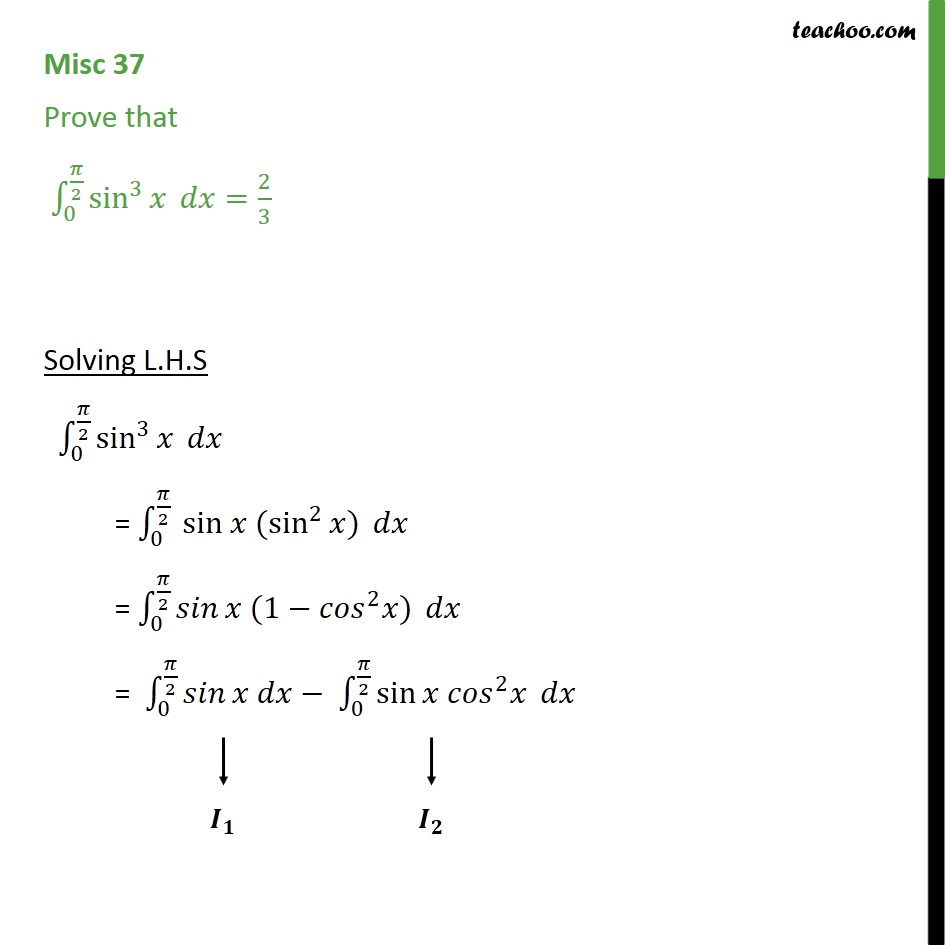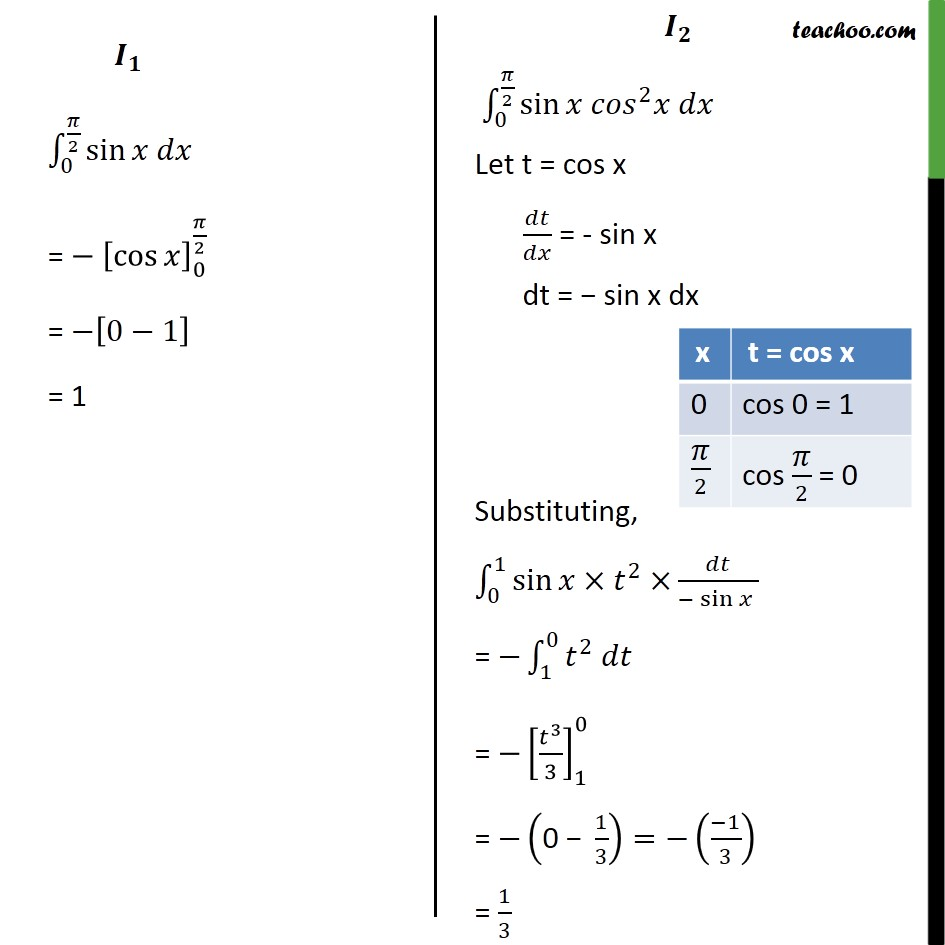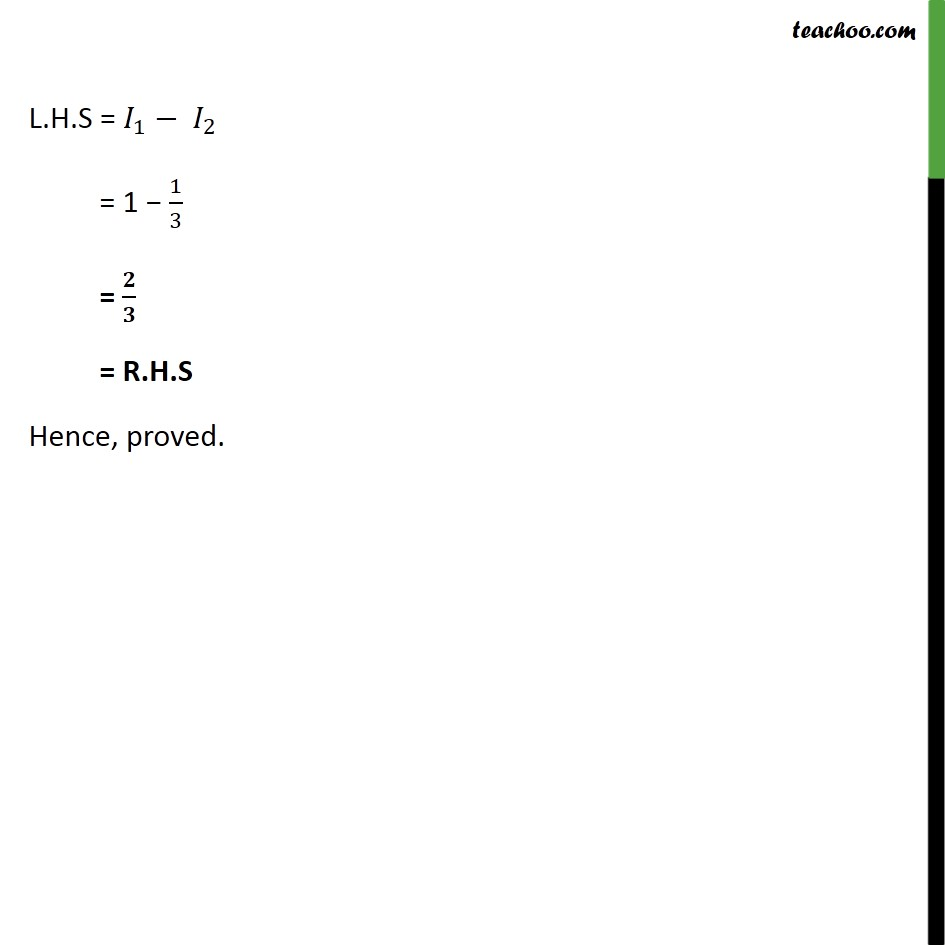1. Chapter 7 Class 12 Integrals (Term 2)
2. Serial order wise
3. Miscellaneous

Transcript

Misc 37 Prove that 0 2 sin 3 = 2 3 Solving L.H.S 0 2 sin 3 = 0 2 sin (sin 2 ) = 0 2 (1 2 ) = 0 2 0 2 sin 2 L.H.S = 1 2 = 1 1 3 = = R.H.S Hence, proved.

Miscellaneous

Chapter 7 Class 12 Integrals (Term 2)
Serial order wise